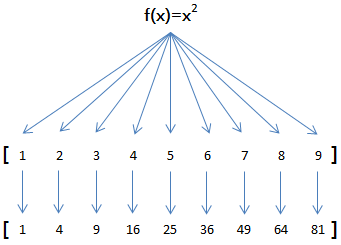# map & reduce

Mr.Hope ... 2019-10-05 JavaScript 大约 6 分钟

# # map & reduce

## # map``````"use strict";

const pow = (x) => x * x;
const arr = [1, 2, 3, 4, 5, 6, 7, 8, 9];
const results = arr.map(pow);

console.log(results); // [1, 4, 9, 16, 25, 36, 49, 64, 81]
``````
1
2
3
4
5
6
7

``````const f = (x) => x * x;
const arr = [1, 2, 3, 4, 5, 6, 7, 8, 9];
const result = [];

for (let i = 0; i < arr.length; i++) result.push(f(arr[i]));
``````
1
2
3
4
5

``````const arr = [1, 2, 3, 4, 5, 6, 7, 8, 9];

arr.map(String); // ['1', '2', '3', '4', '5', '6', '7', '8', '9']
``````
1
2
3

### # reduce

``````[x1, x2, x3, x4].reduce(f) = f(f(f(x1, x2), x3), x4);
``````
1

``````const arr = [1, 3, 5, 7, 9];

arr.reduce((x, y) => x + y); // 25
``````
1
2
3

``````const arr = [1, 3, 5, 7, 9];

arr.reduce((x, y) => x * 10 + y); // 13579
``````
1
2
3

## # filter

filter 也是一个常用的操作，它用于把 Array 的某些元素过滤掉，然后返回剩下的元素。

`map()` 类似，Array 的 `filter()` 也接收一个函数。和 `map()` 不同的是，`filter()` 把传入的函数依次作用于每个元素，然后根据返回值是 `true` 还是 `false` 决定保留还是丢弃该元素。

``````const arr = [1, 2, 4, 5, 6, 9, 10, 15];
const r = arr.filter((x) => x % 2 !== 0);

r; // [1, 5, 9, 15]
``````
1
2
3
4

``````const arr = ["A", "", "B", null, undefined, "C", "  "];
const r = arr.filter((s) => s && s.trim()); // 注意: IE9以下的版本没有trim()方法

r; // ['A', 'B', 'C']
``````
1
2
3
4

### # 回调函数

`filter()` 接收的回调函数，其实可以有多个参数。通常我们仅使用第一个参数，表示 Array 的某个元素。回调函数还可以接收另外两个参数，表示元素的位置和数组本身:

``````const arr = ["A", "B", "C"];
const r = arr.filter((element, index, self) => {
console.log(element); // 依次打印 'A', 'B', 'C'
console.log(index); // 依次打印 0, 1, 2
console.log(self); // self 就是变量 arr
return true;
});
``````
1
2
3
4
5
6
7

``````"use strict";

let r;
const arr = [
"apple",
"strawberry",
"banana",
"pear",
"apple",
"orange",
"orange",
"strawberry",
];

r = arr.filter((element, index, self) => self.indexOf(element) === index);

console.log(r.toString());
``````
1
2
3
4
5
6
7
8
9
10
11
12
13
14
15
16
17

## # sort

### # 排序算法

JavaScript 中 Array 的 `sort()` 方法就是用于排序的，但是排序结果可能让您大吃一惊:

``````// 看上去正常的结果:

// apple 排在了最后:

// 无法理解的结果:
[10, 20, 1, 2].sort(); // [1, 10, 2, 20]
``````
1
2
3
4
5
6
7
8

``````"use strict";

const arr = [10, 20, 1, 2];

arr.sort((x, y) => {
if (x < y) return -1;
if (x > y) return 1;
return 0;
});
console.log(arr); // [1, 2, 10, 20]
``````
1
2
3
4
5
6
7
8
9
10

``````const arr = [10, 20, 1, 2];

arr.sort((x, y) => {
if (x < y) return 1;
if (x > y) return -1;

return 0;
}); // [20, 10, 2, 1]
``````
1
2
3
4
5
6
7
8

``````const arr = ["Google", "apple", "Microsoft"];

arr.sort((s1, s2) => {
x1 = s1.toUpperCase();
x2 = s2.toUpperCase();

if (x1 < x2) return -1;
if (x1 > x2) return 1;

return 0;
``````
1
2
3
4
5
6
7
8
9
10
11

``````const a1 = ["B", "A", "C"];
const a2 = a1.sort();

a1; // ['A', 'B', 'C']
a2; // ['A', 'B', 'C']
a1 === a2; // true, a1 和 a2 是同一对象
``````
1
2
3
4
5
6